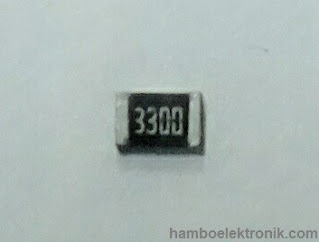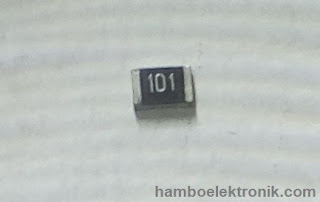How to Calculate a Resistor Color Code Resistor Value

How to Calculate a Resistor Color Code Resistor Value – Previously we have studied the types of resistors. But how do we know the resistance value of the resistor?

So, there are two ways to find out the resistance value of a resistor, friends.

The first is based on the color band and the second value is printed directly on the resistor body.

In this tutorial, I will explain how to read the color band on a resistor. The most basic thing to find resistor color values is to remember the resistor values table.

A. Resistor Value Table

Consider the following resistor color table.Resistor Value Table

B. Consider the following resistor color table.

You have to remember the resistor value table code above. The priority to remember is the Color and Number table because it represents the multiplication column.

Friends, look at the multiplication column, we can replace the multiplication term with "the number of zero".

Friends, look at the color brown, the value is 1. Brown is worth 1, which means that in the bracelet / column the multiplication of zero is one.

Red has a value of 2, zero its two and so on friends and this only applies to the multiplier column.

If you are still confused, here I will give two examples of how we can read the color band on the resistor to find out what the resistance value of the resistor is.

1. First Example

Here in Figure 1 there is a resistor that I provide. This resistor has 4 colored bands on the body of the resistor.Resistor Example 1

Please look back at the color table to see the value of the bracelet color. How to calculate the resistor color is:

Brown, Black, Orange and Gold.
1 ,        0   x  1.000    and  ±5%.
Result: 10.000 Ohm ±5%
(read : 10.000 or 10K Ohm with tolerance ±5%);

2. Second ExampleResistor Example 2

How to read the second example resistor is:

Orange, Orange, Red and Gold.
3,        3   x  100    and  ±5%.
Results: 3.300 Ohm ±5%
(read : 3.300 atau 3K3 Ohm with tolerance ±5%);

In the two examples above are resistors with 4 colored bands, but on the market there are resistors that have 5 to 6 colored bands. Now, for a clearer way to read it, friends can pay attention to the following picture.

Resistor Color Code Chart

In Figure 3 above is a chart of resistor color codes from 4 bands to 6 color bands.

In addition to determining the value of resistance, the color code of the resistor on the 6th band of the color band is also used to determine the temperature coefficient of a resistance.

In English it is called the Temperature Coefficient of Resistance (TCR). The unit of this TCR is ppm / ° C. (parts per million per degree Cetrigrade).

Here are some examples of problems calculating resistor color codes:
• The 1 Ohm Resistor Color Code is brown black gold
• The 100 Ohm Resistor Color Code is brown black brown
• The 1K Ohm Resistor Color Code is Chocolate Red Black
• The Color Code for the 100k Ohm resistor is Brown Black Yellow.
• The black black gold brown resistor is 10 Ohm 5%
• Resistors brown black red gold 102 or 1K Ohm 5%
• Resistors red gold gold gold 2.2 Ohm 5%
• Resistors brown black gold gold 1 Ohm 5%

C. How to Read SMD Resistor Values

It is easier to read SMD resistors than those with colored bands. Figures 4 and 5 are examples of SMD resistors.

Generally, SMD resistors have a value printed on their body. Pay attention to the following picture:

1. First ExampleExamples of SMD resistors read 3,300 Ohm or 3K3 Ohm or 3.3K Ohm

2. Second ExampleExample of an SMD resistor reads 100 Ohms

This is the tutorial on how to read the resistance value on a resistor. If friends have suggestions, feedback or questions, please comment below or contact us.

Thank you for visiting the website Chip Piko. May be useful.